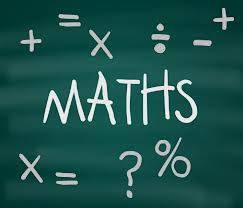# How can I compute the area of a circle, a rectangle and a square?

If you have been asked to calculate the area of any shape, you first need some sort of measurement for each one. For the easiest method for a circle, you will need to measure its radius; for a rectangle, its width and length; and for a square, the length of any one of its sides.The Area of a Circle

For this, you need to measure the radius of the circle. The radius is the length between any point along the edge of the circle to its centre. Let's say the radius is 10cm. Next, you times the radius by itself to find its cube (or put another way, we find the cube of 10). 10 x 10 = 100. Finally, we multiply the cubed radius by Pi (3.1416 to 4 decimal places). 100 x 3.1416 = 314.16cm squared. This is the area of a circle with a radius of 10cm.

The Area of a Rectangle

This is an easier sum than for a circle, but we need two lengths first, the length (one of the two longer sides, which will of course be equal to each other), and the width (one of the two shorter sides, again equal to each other). Once we have these, it's simply a case of multiply one by the other. Say the length is 12 cm and the width is 4 cm. The area would then be: 12cm x 4 cm = 48 cm square.

The Area of a Square

This is even easier! As the length of the sides of the square are all the same, we only need one, and then multiply it by itself. If your square has sides of 14.5cm each, then all we do is: 14.5cm x 14.5cm = 210.25 cm squared in area.

Of course, you may not have been given the measurements necessary for these calculations. Here's some more ideas in that case:

thanked the writer.